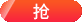由浅入深带你了解PHP表达式

1
2
3
4
5
function foo ()
{
return 5;
}
?>

PHP 和其它语言一样在表达式的道路上发展，但推进得更深远。PHP 是一种面向表达式的语言，从这一方面来讲几乎一切都是表达式。考虑刚才已经研究过的例子，“\$a = 5”。很显然这里涉及到两个值，整型常量“5”的值以及变量 \$a 的值，它也被更新为 5。但是事实是这里还涉及到一个额外的值，即附值语句本身的值。赋值语句本身求值为被赋的值，即 5。实际上这意味着“\$a = 5”，不必管它是做什么的，是一个值为 5 的表达式。因而，这样写“\$b = (\$a = 5)”和这样写“\$a = 5; \$b = 5”(分号标志着语句的结束)是一样的。因为赋值操作的顺序是由右到左的，也可以这么写“\$b = \$a = 5”。

1
2
\$first ? \$second : \$third
?>

1
2
3
4
5
6
7
8
9
10
11
12
13
14
15
16
17
18
19
function double(\$i)
{
return \$i2;
}
\$b = \$a = 5; / assign the value five into the variable \$a and \$b /
\$c = \$a++; / postincrement, assign original value of \$a
(5) to \$c /
\$e = \$d = ++\$b; / preincrement, assign the incremented value of
\$b (6) to \$d and \$e /
/ at this point, both \$d and \$e are equal to 6 /
\$f = double(\$d++); / assign twice the value of \$d before
the increment, 26 = 12 to \$f /
\$g = double(++\$e); / assign twice the value of \$e after
the increment, 27 = 14 to \$g /
\$h = \$g += 10; / first, \$g is incremented by 10 and ends with the
value of 24. the value of the assignment (24) is
then assigned into \$h, and \$h ends with the value
of 24 as well. /
?>

相关阅读